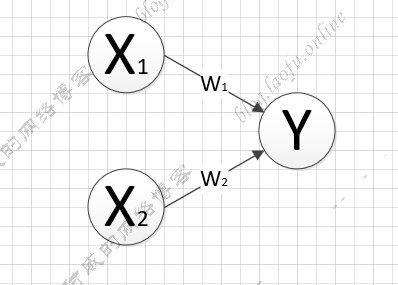# tensorflow的学习笔记--初步认识tensorflow

### 几个概念

TensorFlow是一个基于数据流编程（dataflow programming）的符号数学系统，被广泛应用于各类机器学习（machine learning）算法的编程实现，由谷歌公司开发并开源免费使用。

tensorflow中使用张量代表数据（可以简单理解为参数）,使用计算图来搭建神经网络，使用会话执行计算图，优化对应的权重。

• 张量 多维数组和列表。对于不同维数的张量有不同的名称和表示方法：

标量: 一个数字，比如：1,2,3
向量: 一个数组，[1,2,3]
矩阵: 二位数组，[[1,2],[1,3],[2,3]]
张量: 多维数组

tensorflow的数据的类型很多，与日常编程的数据类型也有点相似之处，先不一一介绍，先看看怎么使用tensorflow（使用pip命令安装对应的依赖模块）

import tensorflow as ts

a=ts.constant([1.0,2.0])# 定义常数
b=ts.constant([3.0,4.0])

result=a+b
print(result)


add表示节点名
0 第0个输出
shape 维度
(2,)一维数组，长度为2
dtype 数据类型

### 简单的模型import tensorflow as ts

x=ts.constant([[1.0,2.0]])# 一行两列
w=ts.constant([[3.0],[4.0]]) # 两行一列

y=ts.matmul(x,w)
print(y)


### 获得运算结果

import tensorflow as ts

x=ts.constant([[1.0,2.0]])
w=ts.constant([[3.0],[4.0]])

y=ts.matmul(x,w)

with ts.Session() as sess:
print(sess.run(y))


tensorflow的学习笔记--初步认识tensorflow

http://blog.laofu.online/2019-03-28-tensorflow-01/

2019-03-28

2019-03-28